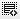## Is there any difference between Gross margin and Profit ?01

Gross Profit is the number
Gross Margin is the %1

Gross margin is the expression of the gross profit as a percentage of sales, whereas the Gross profit is sales minus the cost of sales
Gross Margin= Gross Profit /sales x 100
Gross Profit=sales-cost of sales1

Gross Profit= sales-cost of good sold
Gross Margin(%)= Gross Profit /sales x 1000

gross margin =( gross profit/total revenue)*100 gross profit = totol revenue - cost of goods sold

Click on thisicon to add code snippet.